NCERT Questions

Class 10
Chapter 12 Class 10 - Electricity

## Show how you would connect three resistors, each of resistance 6 Ω, so that the combination has a resistance of (i) 9 Ω, (ii) 4 Ω.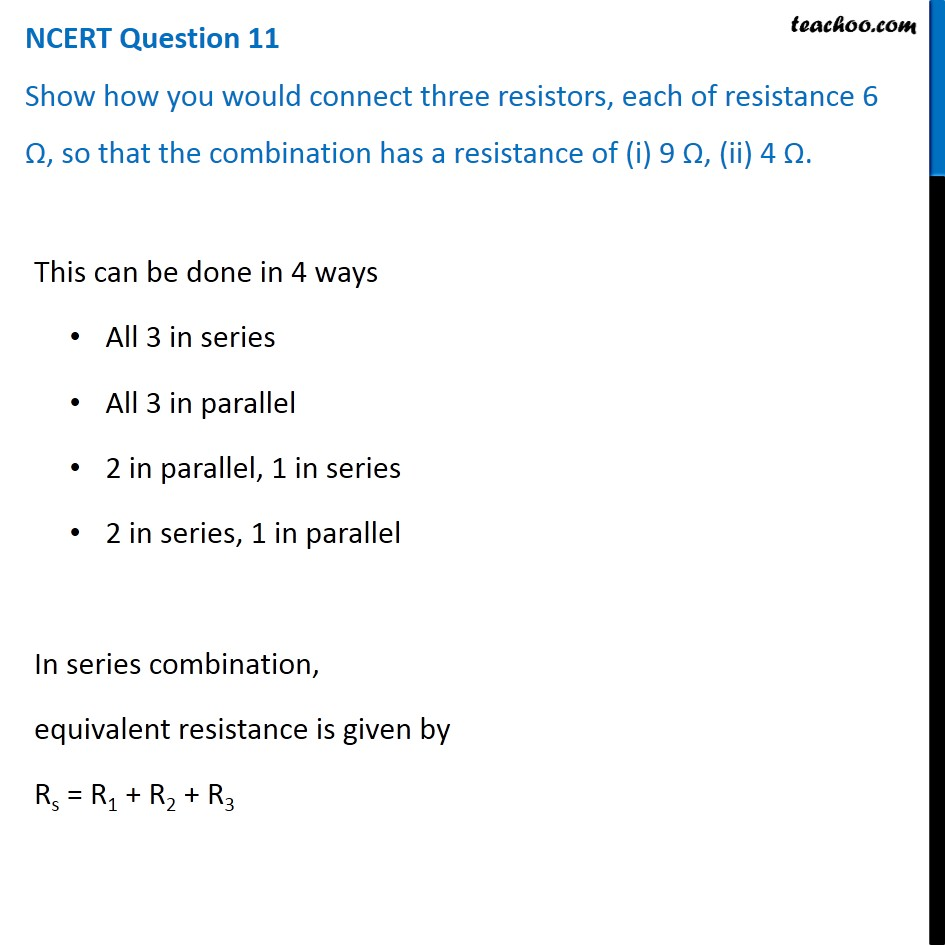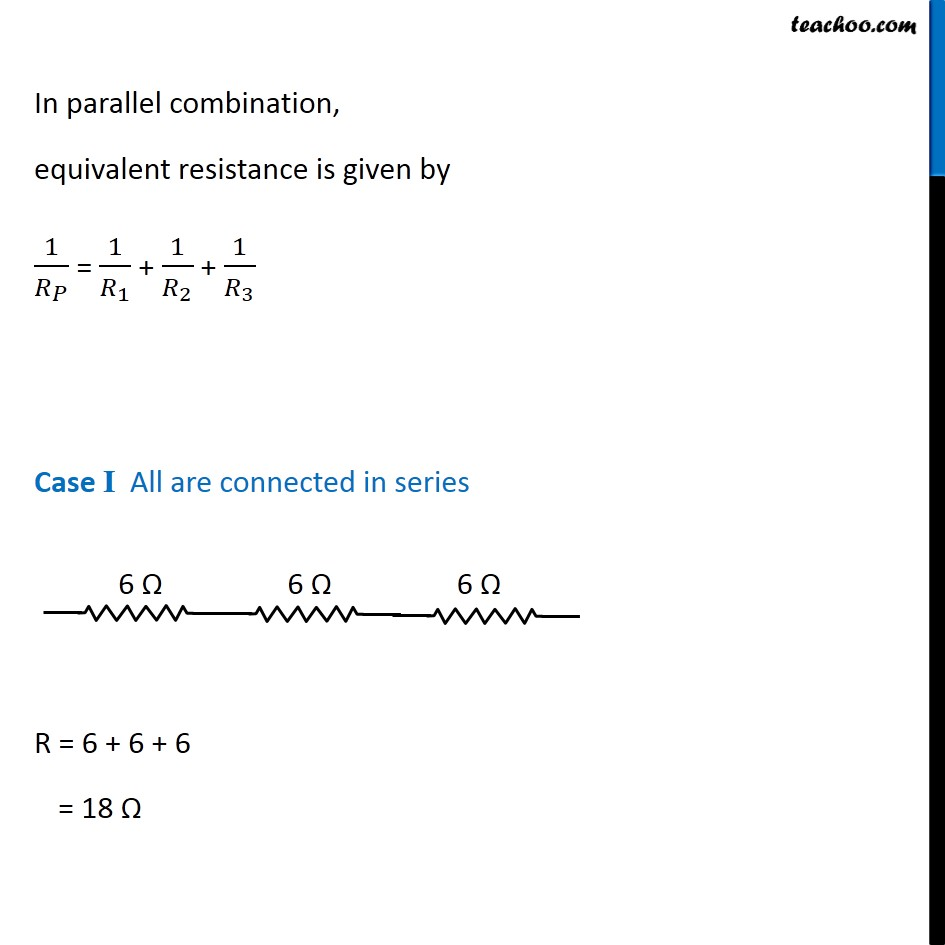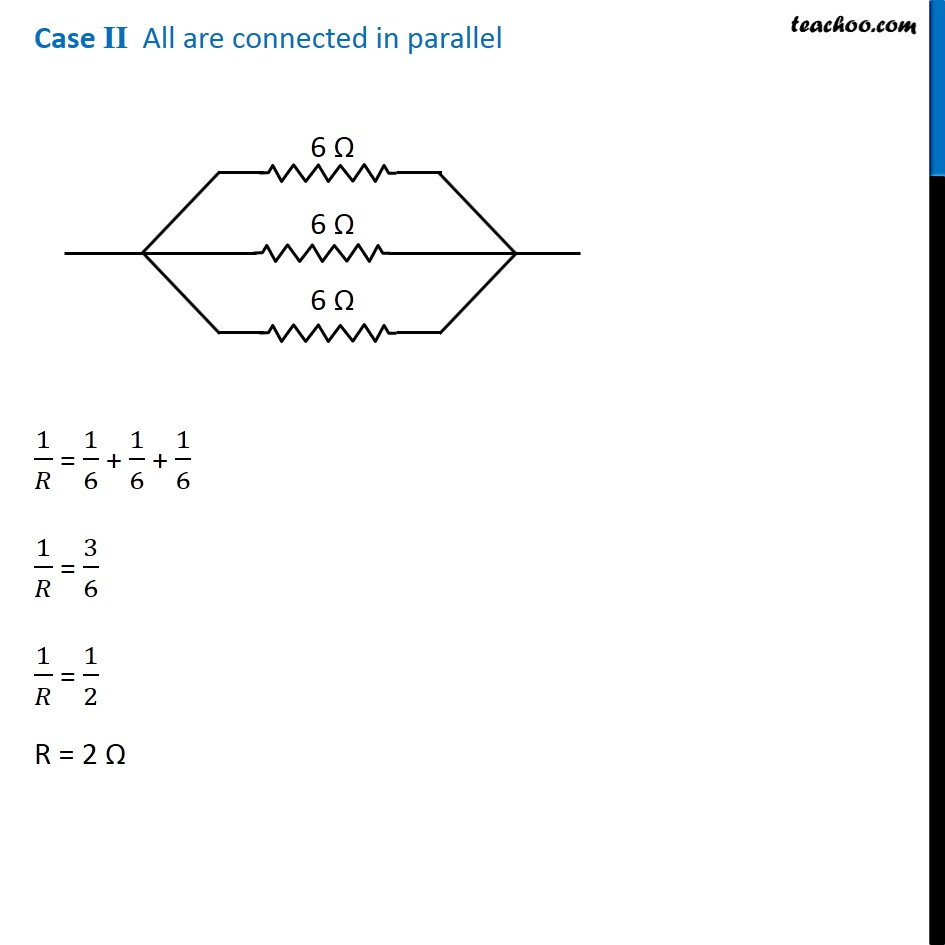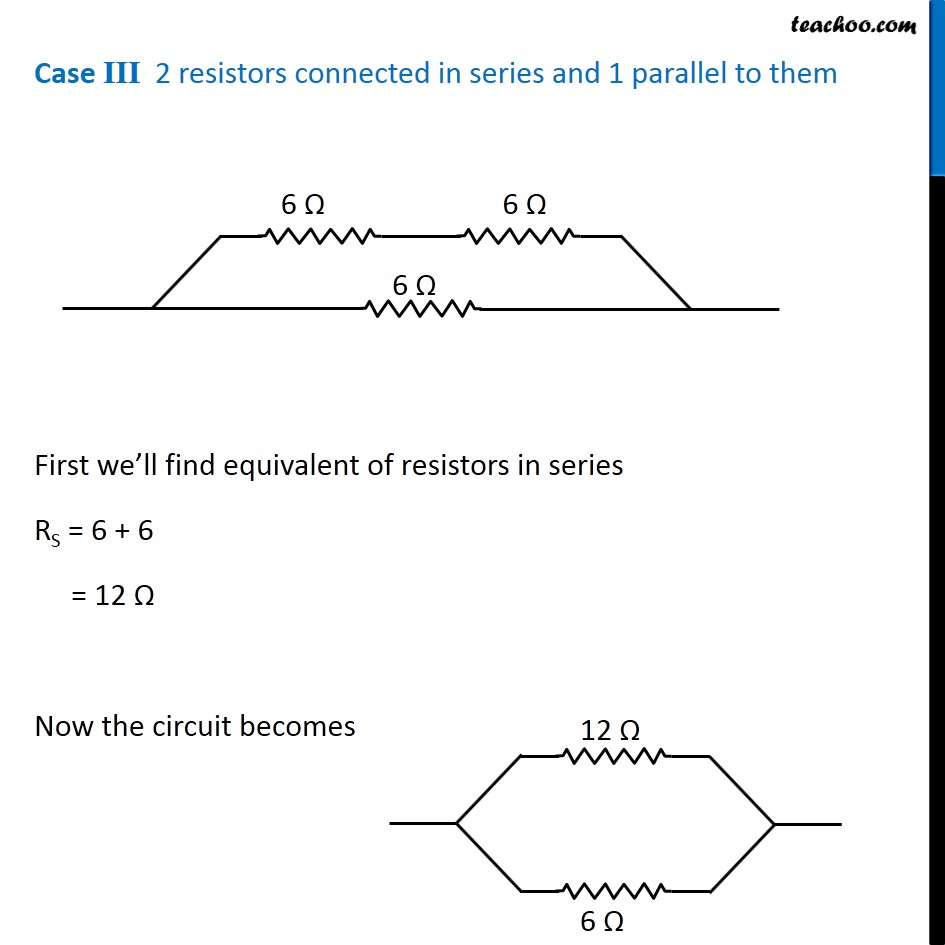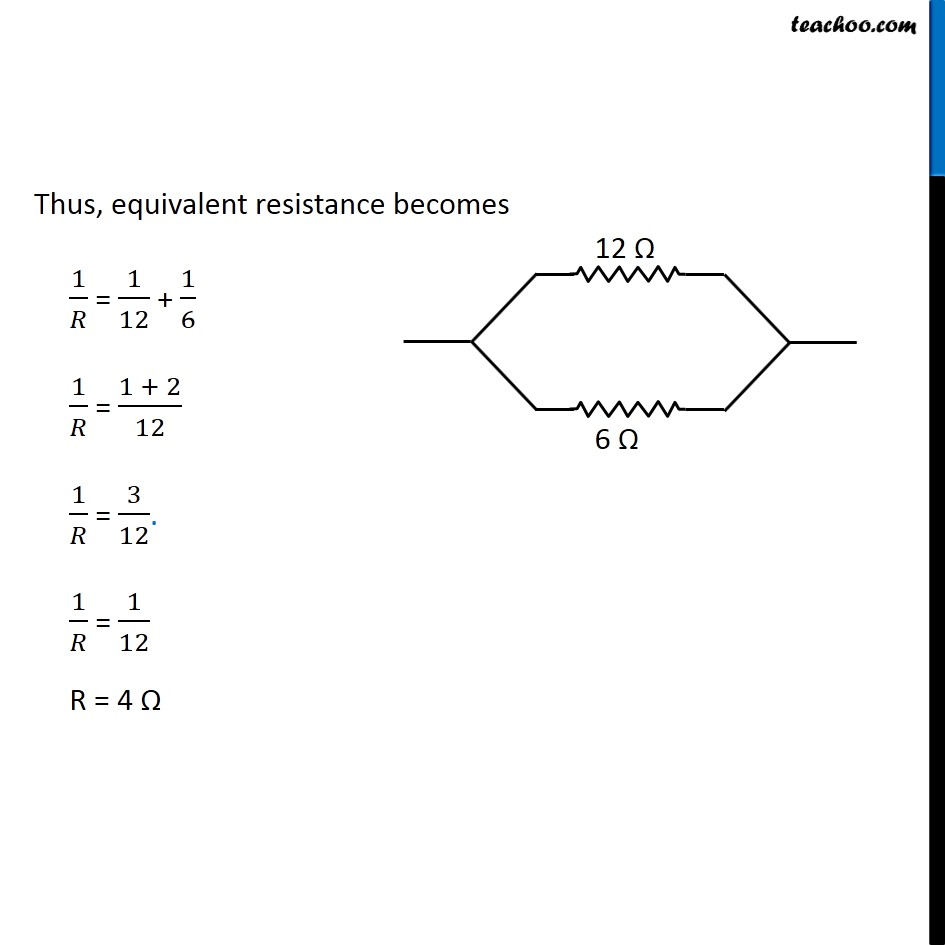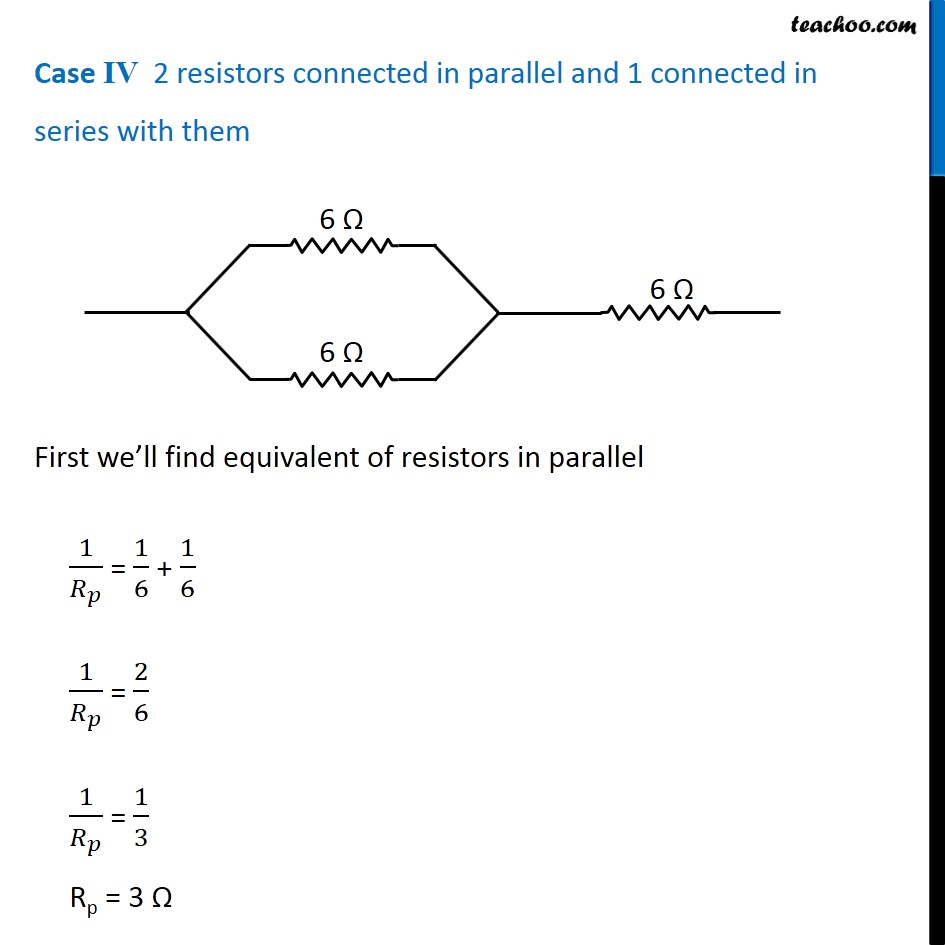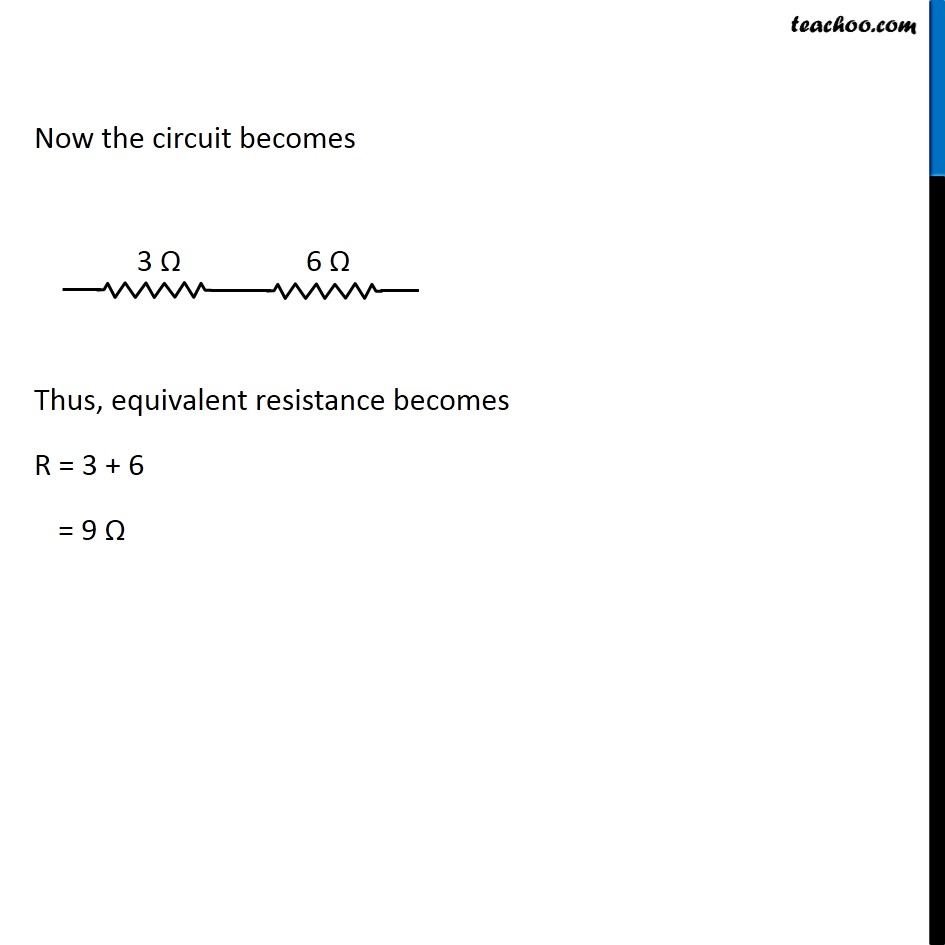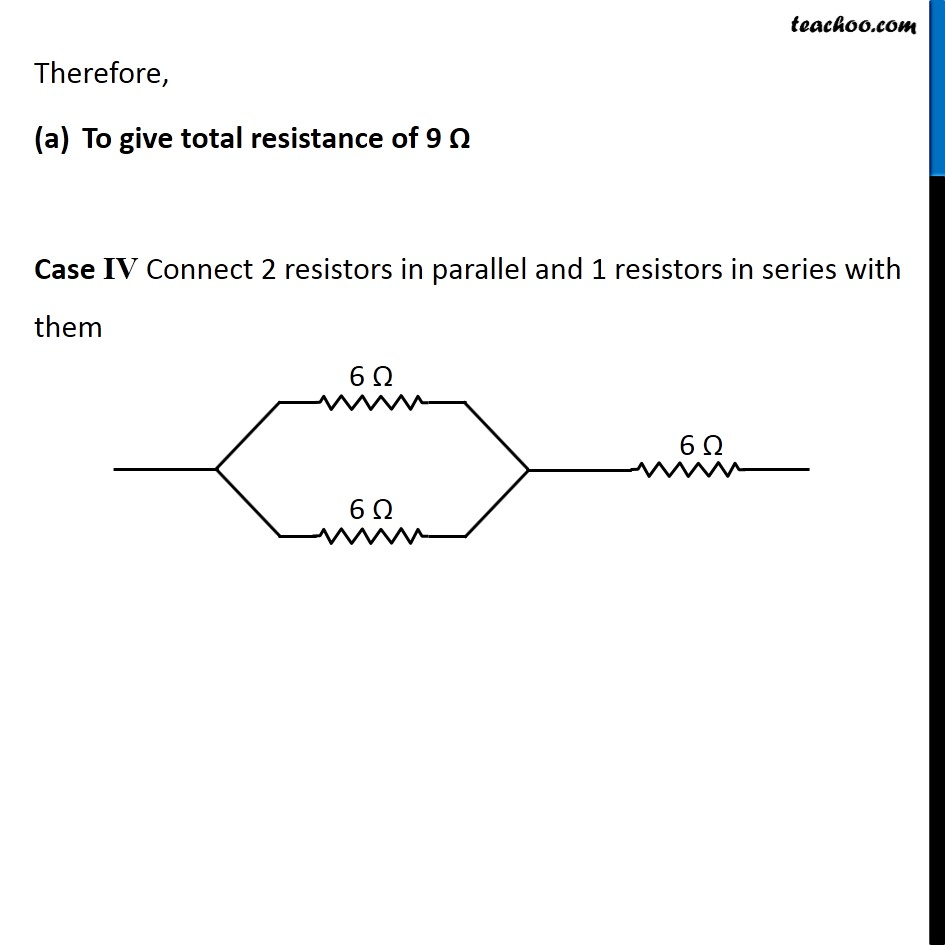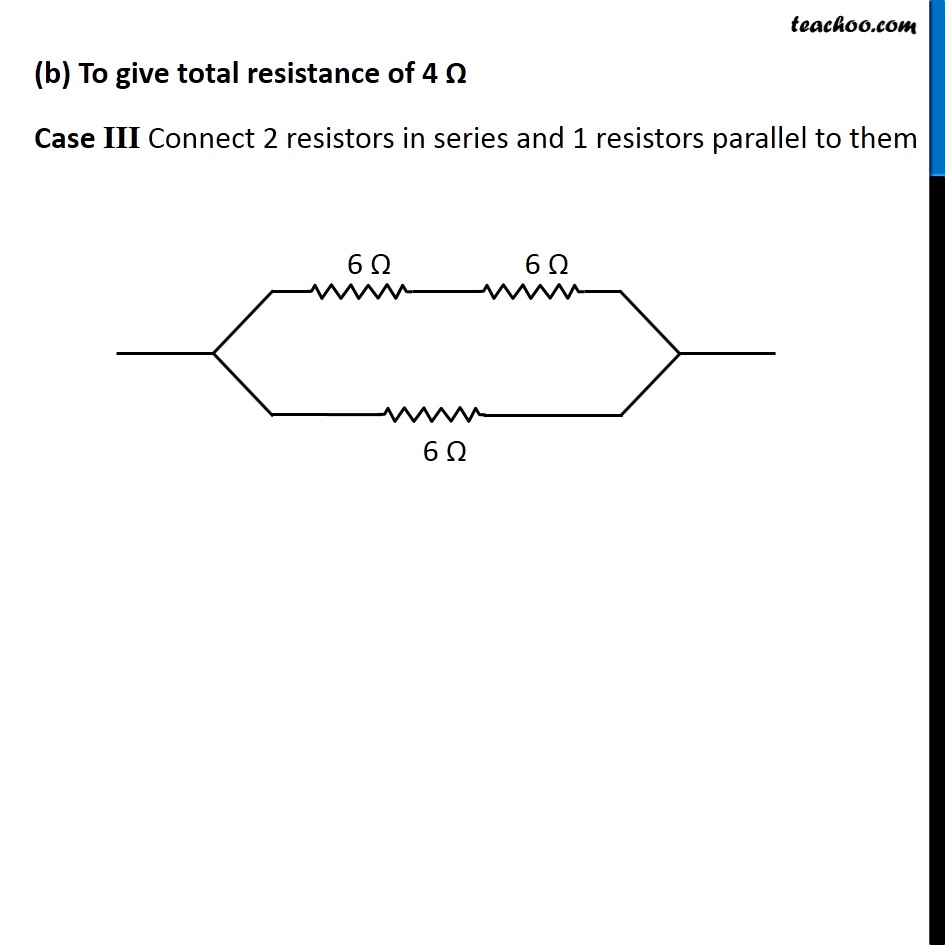Note:- If all 3 resistance were different, we would have 8 cases check

Get live Maths 1-on-1 Classs - Class 6 to 12

### Transcript

NCERT Question 11 Show how you would connect three resistors, each of resistance 6 Ω, so that the combination has a resistance of (i) 9 Ω, (ii) 4 Ω. This can be done in 4 ways All 3 in series All 3 in parallel 2 in parallel, 1 in series 2 in series, 1 in parallel In series combination, equivalent resistance is given by Rs = R1 + R2 + R3 In parallel combination, equivalent resistance is given by 1/𝑅_𝑃 = 1/𝑅_1 + 1/𝑅_2 + 1/𝑅_3 Case I All are connected in series R = 6 + 6 + 6 = 18 Ω Case II All are connected in parallel 1/𝑅 = 1/6 + 1/6 + 1/6 1/𝑅 = 3/6 1/𝑅 = 1/2 R = 2 Ω Case III 2 resistors connected in series and 1 parallel to them First we’ll find equivalent of resistors in series RS = 6 + 6 = 12 Ω Now the circuit becomes Thus, equivalent resistance becomes 1/𝑅 = 1/12 + 1/6 1/𝑅 = (1 + 2)/12 1/𝑅 = 3/12. 1/𝑅 = 1/12 R = 4 Ω Case IV 2 resistors connected in parallel and 1 connected in series with them First we’ll find equivalent of resistors in parallel 1/𝑅_𝑝 = 1/6 + 1/6 1/𝑅_𝑝 = 2/6 1/𝑅_𝑝 = 1/3 Rp = 3 Ω Now the circuit becomes Thus, equivalent resistance becomes R = 3 + 6 = 9 Ω Therefore, To give total resistance of 9 Ω Case IV Connect 2 resistors in parallel and 1 resistors in series with them (b) To give total resistance of 4 Ω Case III Connect 2 resistors in series and 1 resistors parallel to them